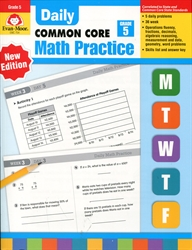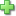# Daily Math Practice Grade 5

Price: \$22.99

See series description for full review.

The skills practiced in Daily Math Practice, Grade 5 include:

Computation

• multidigit addition with and without regrouping
• multidigit subtraction with and without regrouping
• multidigit multiplication and division
• addition, subtraction, multiplication, division of decimals and fractions

Number

• base ten system
• word/standard forms
• place value
• rounding
• estimation
• properties/relationships
• and much more

Patterns/Algebra

• figural patterns
• numerical patterns
• expressions
• function tables
• equations

Geometry/ Spatial

• 2-dimentional shapes
• 3-dimentional shapes
• symmetry
• congruency
• spatial
• angle

Measurement

• weight
• capacity
• time
• temperature
• and much more

Data/Probability

• coordinate graphing
• constructing graphs
• interpreting graphs
• range
• mode
• and much more
Did you find this review helpful?
Series Description
Related Categories
Recommended for...
Customer ReviewsClick here to write a review